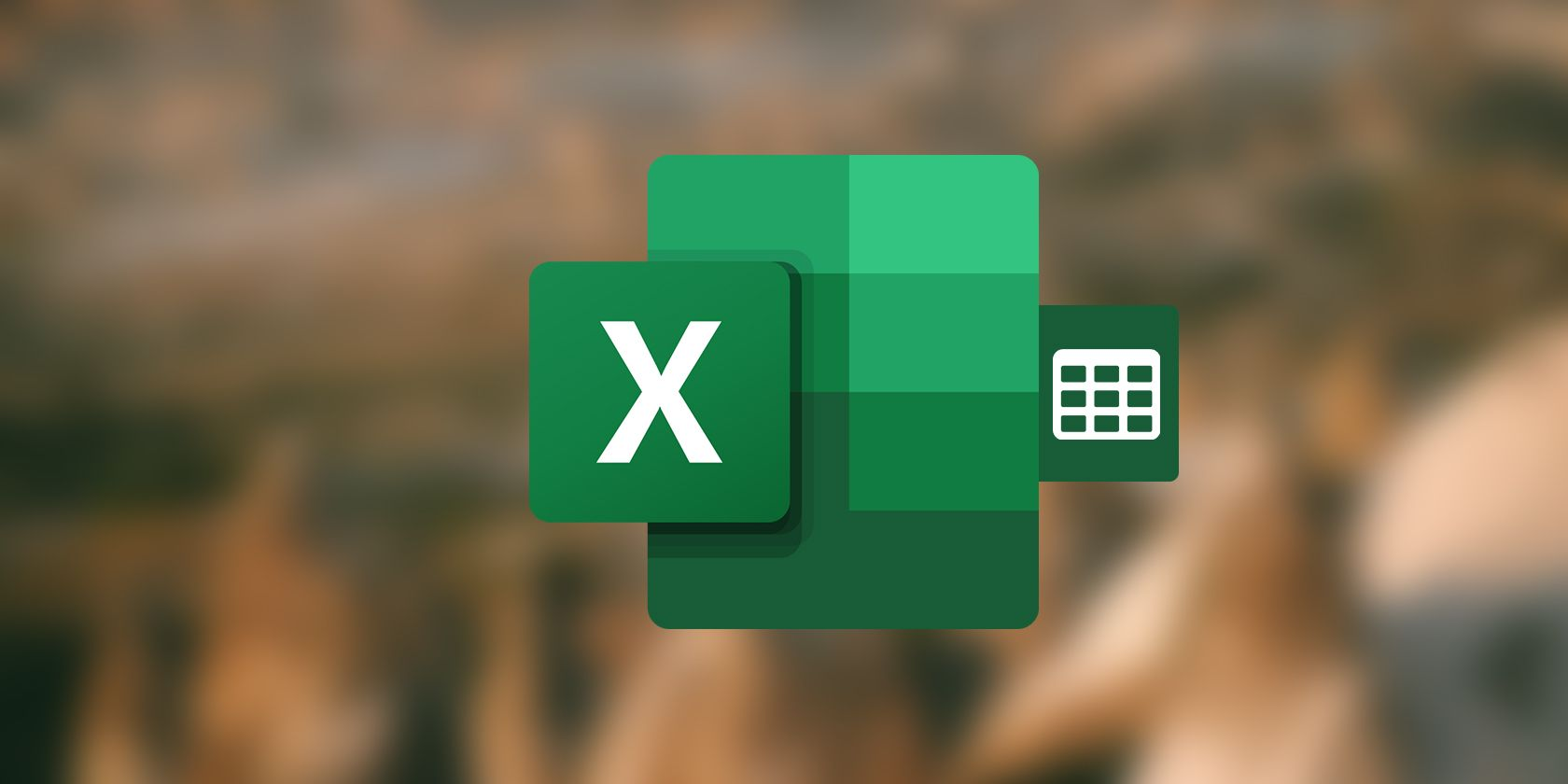# How to Create a Table in Excel

Excel tables can quickly give the data in your spreadsheet an organized look and make them easier to manage. Once you format a range of cells as an Excel table, you’ll be able to sort and filter the data in them, and use structure references in related formulas.

Creating an Excel table is an easy task, but despite this, Excel tables are a cornerstone of many spreadsheets. Read on to find out how you can create these handy tables in Excel!

## What Are Tables in Excel?

Even though an Excel spreadsheets look like big tables, a range of cells is not considered an Excel table until you format them as such. Excel tables consist of a header, which is usually the first row in the table, and the columns under the headers. You can use the header row to filter the data in your Excel table.

Once you format your range of cells as a table, not only you will be able to easily sort and filter the data using the headers, you can also use structure references in the formulas. Structure references are references made to a structure in the table, like a row or a column, rather than a cell.

A formula using structure references can output the results to a structure in the table, so that multiple cells are affected by a single formula. Though this might sound similar to array formulas, the output of a formula with structure references isn’t necessarily an array.

Excel tables are similar to Excel data tables in name, but these two are very different. Data tables are a part of Excel’s What-if analysis tool kit. You can learn more about Data tables in our article on how the data table in Excel lets you compare results.

## How to Create a Table in Excel

Despite its importance, creating a table in Excel is simple. In this example, we have the sales that a number of employees have made in two quarters. The goal is to format the data as an Excel table, and then to calculate the total sales using the SUM function in Excel with structure references.

1. Select your range of cells. In this example, that is A1: D11.
2. In the Home tab, click on Format as Table in the Styles section.

3. Select a style for your table. Note that if your cells already have styling, then the table style will be ignored.
4. Check My tables has headers if you have already put out headers. If not, you can uncheck this option so that Excel automatically adds headers. We’re going to keep this checked.

5. Click OK.

Voila! You have an Excel table! You can now use the headers to sort and filter your data in any manner you see fit. However, our job here isn’t done yet. It’s time to create a formula to calculate the total sales for each employee:

1. Select the first cell where you want to display the formula results. That’s going to be cell D2 in this example.
2. In the formula bar, enter the formula below:
``=SUM(Table1[@[Q1 Sales]:[Q2 Sales]])``

It’s best if you type a formula containing structure references, rather than paste it, since the headers are unique. Once you type the at sign after the bracket, Excel will prompt you with the available headers in Table1. In this formula, we’ve called on the SUM function and made structure references to Q1 Sales and Q2 Sales column. As a result, this formula will sum the numbers in these two columns together.

3. Press Enter.

Once you press Enter, Excel will instantly sum the columns and display the results. Note that since you used a reference structure, all the cells were filled automatically.

## Excel Tables Make Analysis Easier

Excel is a powerful spreadsheet app intended to help you with organizing and analyzing data. Excel tables do exactly that for you, they organize the data and make analysis easier. Using the headers in your table, you can sort and filter your data with just a couple of clicks, and without having to use functions.

Excel tables also grant you the perk of using structure references in your formulas, which can make calculations in mass scale much simpler. Now that you know how to create and use Excel tables, it’s time to put this new-gained skilled to use in your spreadsheets!